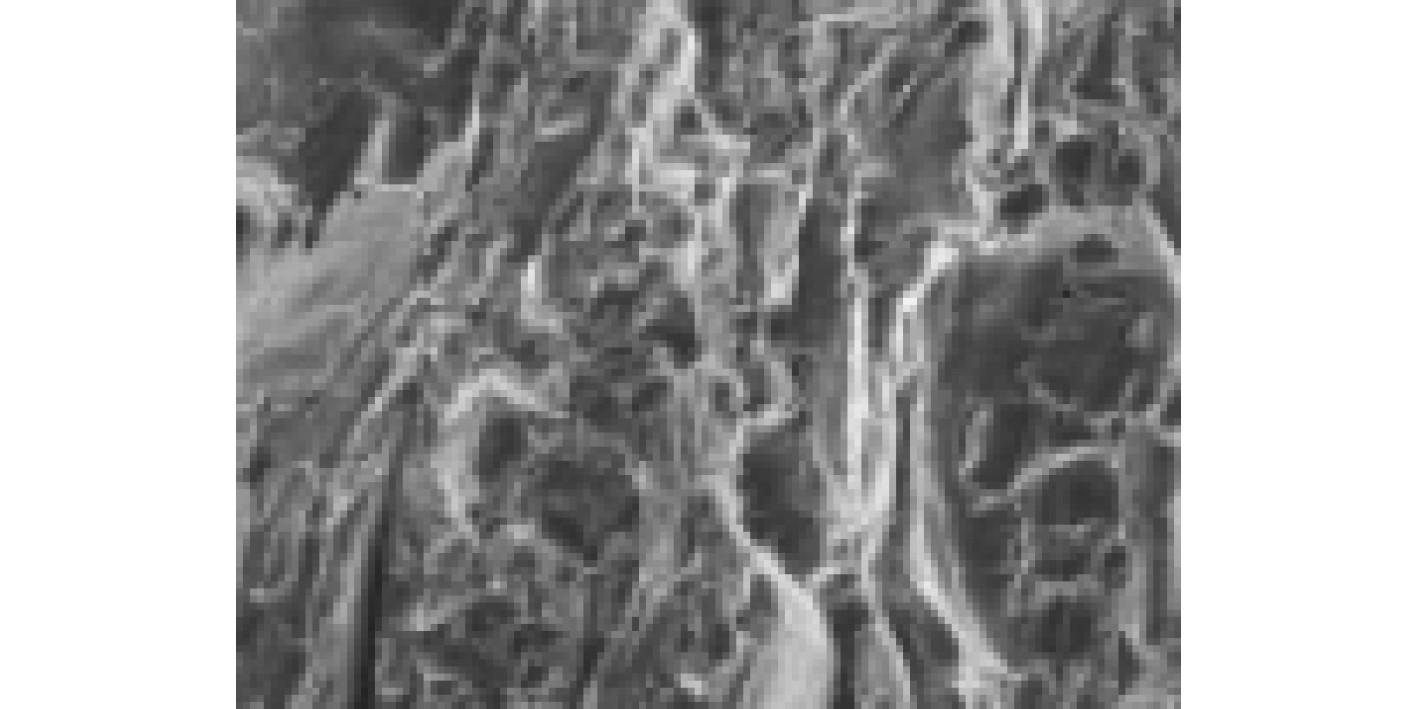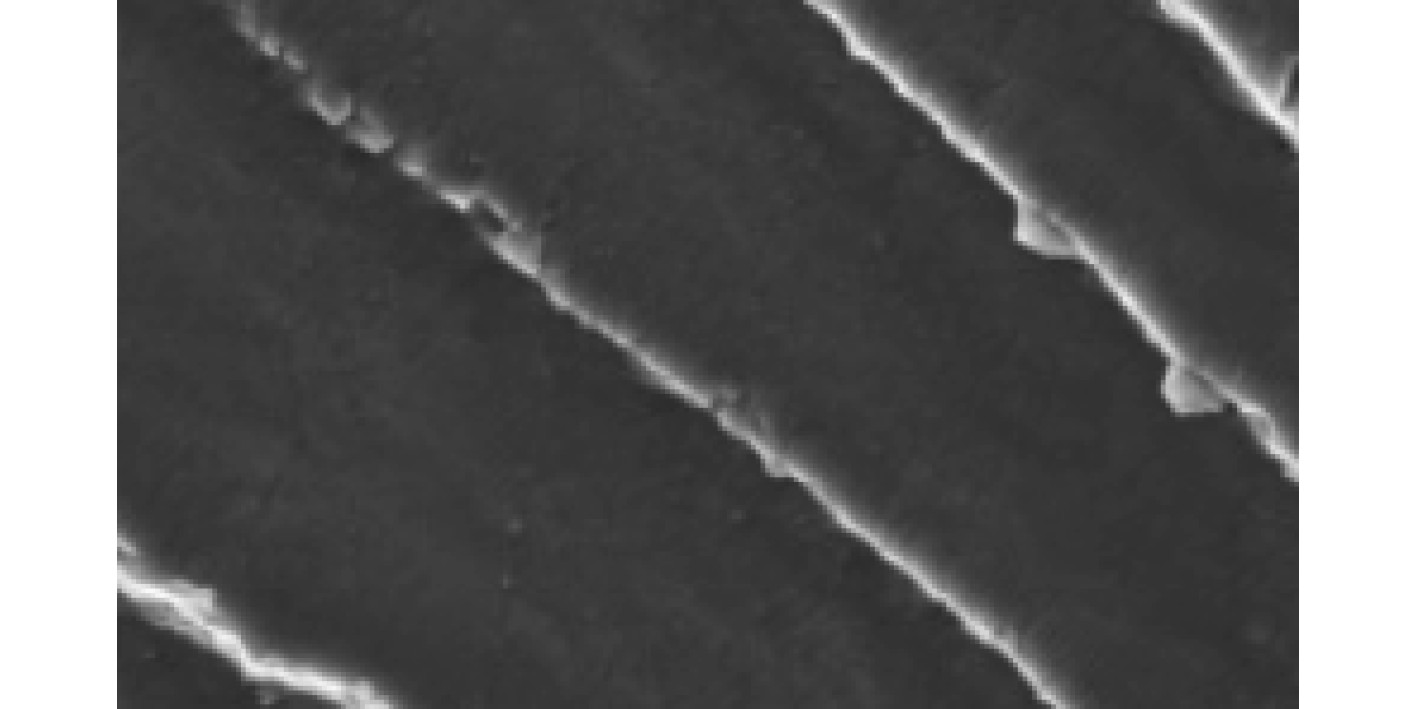﻿ 螺旋桨激励下舰船外板机械疲劳寿命预测准确度分析
 舰船科学技术2023, Vol. 45Issue (1): 41-44    DOI: 10.3404/j.issn.1672-7649.2023.01.008PDF

Analysis of prediction accuracy of mechanical fatigue life of ship outer plate under propeller excitation
ZHANG Song-qi, LI Yuan-yuan
Hebi Instiute of Engineering and Technology, Henan Polytechnic University, Hebi 458030, China
Abstract: Mechanical fatigue is the main cause of the damage of the hull structure. Both military and civilian ships, there is the problem of fatigue. There have been many maritime accidents around the world, and the problem caused by mechanical fatigue has become the concern of the classification societies around the world. At present, ships are developing towards high speed and large scale, and the performance of its single machine has been rapidly improved. However, due to the restriction of many factors, the diameter of the propeller cannot be increased too large, resulting in the load of the propeller and the excitation force of the propeller. In view of the cracks in the outer plate of many high-speed ships after one to six months, this problem is analyzed in this paper.
Key words: mechanical fatigue     propeller     ship
0 引　言

1 疲劳破坏的基本原理图 1 微观裂纹机械疲劳扩展 Fig. 1 Microscopic crack mechanical fatigue extension图 2 机械疲劳裂缝 Fig. 2 Mechanical fatigue crack

1.1 S-N曲线法疲劳分析

S-N曲线法是以S-N曲线和Palmgren-Miner线性累积损伤判据为基础，机械疲劳破坏导致整体寿命变短，这是通过循环应力或（塑性或整体）应变来描述的。其中，在没有出现初始裂缝（表面名义上是平滑的）的情况下，对试验样品进行应力振幅或应变振幅的控制，这样得到的使用寿命包含了引起主要裂缝的疲劳周期（可能达到90%的全部疲劳寿命）和疲劳周期的数目，这些疲劳周期会造成主要裂缝的突然破裂。从理论上讲，S-N曲线方法只能用于裂纹初始寿命的预报，而目前“疲劳误差”这个概念往往被人为地定义为“失效”，采用S-N曲线方法对结构进行全寿命估计，从而简化了计算。

1.2 断裂力学方法

S-N曲线法采用“损伤容限”原理进行疲劳分析，在此，最重要的是所有的技术部件都是固定的。一般通过非破坏性的检查，如视觉、染色、X射线、超声波、磁或声发射等。若元件没有受到损伤，则应该进行可靠性试验，例如在电压等级稍高的情况下，对压力容器等大型的结构进行仿真试验。在非破坏性试验中没有发现裂缝，而在可靠性试验过程中没有出现突然的破坏。

1.3 可靠性方法

2 螺旋桨激励确定 2.1 估算公式适应性

2.2 螺旋桨激振力的理论计算

 $P + \frac{{{u}}_{\text{t}}^2}{2} + \rho \frac{{\partial \varphi }}{{\partial {\text{t}}}} = C \text{，}$

 $P = - \rho \frac{{\partial \varphi }}{{\partial {{t}}}} + \rho \nu \frac{{\partial \varphi }}{{\partial {{x}}}} \text{，}$

 $\begin{split} \varphi =& \sum\limits_{{{s}} = 1}^{{z}} {\sum\limits_{{{n}} = {{0}}}^{{s}} {{\varphi ^{{x}}}} }=\\ &\sum\limits_{{{s}} = 1}^{{z}} {\sum\limits_{{{n}} = {{0}}}^{{s}} {{\varphi ^{{x}}}} } {\text{sin}}\left( {{{n}}{\nu _{{s}}} + {{\left( {{\omega _{{n}}}} \right)}_{{n}}}} \right) 。\end{split}$

 ${\nu }_{\text{s}}=\nu +{s}\times 2{\text{π}} /Z text{，}$
 $\varphi = \sum\limits_{s = 1}^z {\sum\limits_{n = 0}^s {\varphi _{kz}^x} } Z\sin \left( {kZ\nu + {{\left( {{\omega _n}} \right)}_{kz}}} \right) 。$

 $\begin{split}{l}p=&-\rho \displaystyle \sum _{{k}=0}^{\infty }\Biggr({\phi }_{kz}^{x}k{Z}^{2}2{\text{π}} n{\cos}(2{\text{π}} nkzt+({\omega }_{n})_{kz})-\\ &\nu \dfrac{\partial {\phi }_{{kz}}^{{x}}}{\partial \text{x}}Z{\sin}(2{\text{π}} nkzt+({\omega }_{{n}})_{{kz}})\Biggr) 。\end{split}$

3 板的有限元分析 3.1 板的有限元列式建立

3.2 板的位移场

 $\begin{array}{l}u(x，y，z)=u_{0}(x，y)+z{\theta }_y，\\ \nu (x，y，z)={\nu }_{0}(x，y)-z{\theta }_x，\\ w(x，y，z)=w_0(x，y)。\end{array}$

4 机械疲劳寿命预测 4.1 疲劳的破坏特征

4.2 疲劳寿命预测的研究方法

 $\sum {\frac{{{n_i}}}{{{N_i}}}} = 1。$

 $\frac{{\Delta {{w}}}}{{{W}}} = \frac{1}{{{N_{{i}}}}} 。$

 $\sum {{w_i}} = W \text{，}$

 $\sum {\frac{{{{{w}}_{{i}}}}}{W}} = \sum {\frac{{{{{n}}_{{i}}}}}{{{{{N}}_{{i}}}}}} = 1。$

Miner判据的成功之处在于，经过了大量的实验验证，在工程上容易应用。

4.3 影响疲劳累积损伤的重要因素

5 结　语

  李斌, 于文太, 樊鹤, 等. 基于LQG算法和复合形法的深水铺管船动力定位数值模拟[J]. 中国海洋平台, 2020, 35(6): 46-51. LI Bin, YU Wen-tai, FAN He, et al. Numerical simulation of dynamic positioning of deepwater pipe-laying vessel based on LQG algorithm and composite shape method[J]. China Offshore Platform, 2020, 35(6): 46-51.  李勇跃, 毋晓妮, 张良, 等. 海上油田溢油回收船动力定位能力与艏部推进器配置优化[J]. 船舶设计通讯, 2020(2): 17-21. LI Yong-yue, WU Xiao-ni, ZHANG Liang, et al. Optimization of dynamic positioning capability and bow thruster configuration of offshore oil spill recovery vessel[J]. Ship Design Communication, 2020(2): 17-21. DOI:10.3969/j.issn.1001-4624.2020.02.005  薛龙玉. 船舶动力系统的绿色转型——访中船动力集团首席技术总监吴朝晖[J]. 中国船检, 2022(3): 34-37. XUE Long-yu. Green transformation of ship power system——Interview with Wu Zhaohui, Chief Technical Director of CSSC Power Group[J]. China Ship Inspection, 2022(3): 34-37. DOI:10.3969/j.issn.1009-2005.2022.03.010  林文锦, 张亚东, 安志辰. 海洋石油支持船动力定位模块推力分配策略[J]. 集美大学学报(自然科学版), 2022, 27(4): 353-358. LIN Wen-jin, ZHANG Ya-dong, AN Zhi-chen. Thrust distribution strategy of dynamic positioning module of offshore oil support vessel[J]. Journal of Jimei University (Natural Science Edition), 2022, 27(4): 353-358.  赵亮, 汪学锋, 徐胜文, 等. 考虑内孤立波的钻井船动力定位能力分析[J]. 中国海洋平台, 2020, 35(5): 35-40+46. ZHAO Liang, WANG Xue-feng, XU Sheng-wen, et al. Analysis of drilling ship dynamic positioning capability considering internal solitary waves[J]. China Offshore Platform, 2020, 35(5): 35-40+46.